Ultrafast Electron Plasma Index: An Ionization Perspective | OMICS International
Journal of Lasers, Optics & Photonics

# Ultrafast Electron Plasma Index: An Ionization Perspective

Arissian L1,3* and Diels JC1-3

1Electrical and Computer Engineering, University of New Mexico, USA

2Physics and Astronomy, University of New Mexico, Albuquerque, New Mexico, USA

3Center for High Technology Materials, Albuquerque, New Mexico, USA

*Corresponding Author:
Arissian L
Electrical and Computer Engineering
University of New Mexico, USA
Tel: 505-5077058
E-mail: [email protected]

Received Date: April 03, 2014; Accepted Date: May 23, 2014; Published Date: May 29, 2014

Citation: Arissian L, Diels JC (2014) Ultrafast Electron Plasma Index: An Ionization Perspective. J Laser Opt Photonics 1:107. doi:10.4172/2469-410X.1000107

Copyright: © 2014 Arissian L, et al. This is an open-access article distributed under the terms of the Creative Commons Attribution License, which permits unrestricted use, distribution, and reproduction in any medium, provided the original author and source are credited.

Visit for more related articles at Journal of Lasers, Optics & Photonics

#### Abstract

In the case of gases weakly ionized with ultra-short pulses, the electrons that follow the laser field are neither bound nor free. This review paper presents a simple approach to calculate the electron index based on a known electron motion in a non-relativistic regime. This basic approach does not neglect the time varying index. This review is an effort to capture concepts of the transient electron index, like the electron index created in the laser filamentation.

#### Keywords

Ionization; Ultra-short pulses; Laser filamentation

#### Introduction

The index of refraction seems a trivial subject that we have been introduced to since the first optics courses, through Snell’s law. In the middle of the twentieth century there were a few attempts to have a better understanding of the index of refraction. With a classical mechanics picture in mind, the derivation of the index was a combination of the principle of least action and Fermat’s principle. In the current textbook approaches, the index of refraction is calculated by solving a damped harmonic oscillator for an electron set in motion by an applied electric field “E”. The two main classes of bound and free electrons are considered such that the motion of the first is 180 degree out of phase with the second. As a result free electrons create a negative index whereas bound electrons a positive one. In a general case the motion of the electrons may not be an harmonic oscillator motion, or it could be driven by multiple electric fields. In the case of gases weakly ionized by ultra-short pulses, the electrons that follow the laser field are neither bound nor free. This review paper presents a simple approach to calculate the electron index based on a known electron motion in a non-relativistic regime. The aim of this work is to collect some known facts about the index of refraction of the weakly ionized air. All the concepts mentioned in this work had been studied in more details elsewhere but had not been collected in one manuscript to address the mutual interaction of light and low density photo-ionized electrons.

The gas under consideration is air at atmospheric pressure and room temperature, multiphoton or tunnel ionized by ultrashort optical pulses. The pulses are considered Gaussian in time and space with 200fs duration [Full Width at Half Maximum (FWHM) of the intensity]. The optical beam is a non-diffracting laser filament. In the case of high intensity filamented beams [1-9], the beam profile can be reasonably approximated by a Gaussian, as has been measured in the case of 800 nm filament  and demonstrated by theory and experiment at 266nm . Other non-diffracting spatial intensity profiles may exist, as a Bessel beam profile created by axicon type focusing [5,12]. The beam width is typically 200 micron FWHM, when the peak intensity of the ultrashort pulse is clamped at a value of 5×1013 Watt/cm2  for 800 nm filaments. In both UV and 800nm filaments, the total ionization rate of the air is 10-3 to 10-4. The traditional calculation of index of refraction is based on the Drude model . The notion of free electrons in the Drude model implies an ensemble of electron repelling each other, a mutual interaction responsible for a restoring force, which is at the origin of the plasma resonance. Density fluctuations of a cloud of electrons have a resonance at a frequency ωp proportional to the square root of the electron density.

For an optical frequency above that frequency, the negative contribution of the index of refraction of the plasma can be shown to be proportional to the square of plasma frequency (about 1 THz for the density of 1016 cm-2 corresponding to the ionization rate in the filament). It is that negative contribution to the index that ensures that the filament in air maintains its size beyond the Rayleigh range, by balancing the positive nonlinear Kerr focusing .

This model of balance between opposite indices of refraction of Kerr effect and plasma is a clear oversimplification. During the life time of a laser pulse of 200fs the plasma is not in equilibrium and its index should be time dependent. It is also conceptually disturbing to consider a balance between nonlinear Kerr focusing which follows the laser pulse with a steady state Drude index that is constant and defined by the density of the electrons created over the pulse length. In the short time scale before plasma equilibrium the motion of the electrons (hence their radiation) is not determined by their density (the concept of plasma frequency emanates from Coulomb repulsion between charges). The electrons are driven by the laser field, and through their motion create a field that adds to the laser field. The emphasis of this work is to calculate the contribution of ultrafast electron motion in a weakly ionized air (single ionization) to the (time dependent) index of refraction. To highlight this effect, all other interactions such as electron-electron, electron-neutral and electron with its parent ion are neglected, which is justified in a time short compared with the plasma period. The motion of the electron is non-relativistic (v/c<100).

For the purpose of this study the electron index is calculated as a radiation response of charges in an infinitesimal cross section of the beam. The index of refraction ne(t, r) as a function of position and time can be combined with propagation codes [15-17]. The laser pulse length is short enough (200 fs) to neglect electron-electron collisions and the ion’s motion. The laser pulse is long enough to neglect influences of carrier to envelope offsets (the change in peak field is small from one oscillation to the next).

Ionization

Let us choose for the optical pulse a Gaussian in time and space with field description of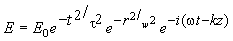where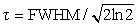and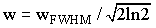are related to their practical measurement of FWHM of intensity in time and space. High energy Gaussian laser pulses induce dipole oscillation in neutral molecules. The nonlinear response of these bound electrons (Kerr effect) will result in a positive refractive index  that is stronger on the beam axis. This positive Kerr focusing, with a fast electronic and slower molecular component, results in an increase of intensity on axis. As the field intensity increases, so does the probability of tunnel ionization. The resulting free electron production has a negative refractive index with its strength proportional to the number of electrons, hence the optical field. As a result one can attribute a negative lens effect to the electrons, which combines with the divergence due to diffraction to balance the positive Kerr focusing of response. This balance can be maintained over a few Rayleigh ranges for IR pulses, when the initial pulse power is above the critical power (3.2 GW for air at 800 nm, and 13.5 MW at 266 nm ) [19,20]

The two channels of strong field ionization of air molecules are distinguished by the Keldysh parameter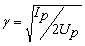where “Ip ” is the ionization potential and Up is the ponderomotive energy, average kinetic energy of a free electron oscillating with the laser field. In the “quasistatic limit” of γ<1 the dressed Coulomb barrier is essentially static as seen by the electrons and the method of releasing the electrons is dominated by tunneling. For γ>1 the electron release is most likely described by photon absorption, and multiphoton features are more dominant . For the filament condition in this study, even though the Keldysh parameter is almost unity, the tunneling features of ionization have been observed . For the purpose of this study the modified ADK tunneling rate is considered .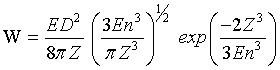(1)

in which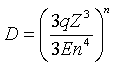where “q” is the electron charge, “Z ” is the charge of atomic or ionic core (one in the present case where we consider only single ionization),“n”is the effective principal quantum number defined through Rydberg formula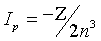(Ip being the ionization potential or binding energy used in Keldysh parameter above). The relation between the ionization rate with linearly polarized light Wl to circularly polarized light Wc is [25,26]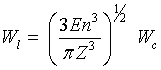(2)

For the higher laser intensities, in the case of barrier suppression ionization, the ionization rate is no longer polarization dependent. The yield at each time “t” is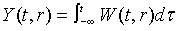(3)

The density of electrons is N (t) = Y (t)Na , where “Na” is number density of neutral molecules. The density, hence the spacing R(t) between electrons, is modified with time: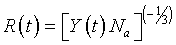(4)

The density of dry air (20°C) is 2.504×1025 m-3 which gives a mean free path of R=N-1/3 of 3.42×10-9 m. For 10-3 ionization probability the mean free path of the electrons is 34.2nm.

As the electron leaves the tunnel at time t0, its motion is defined by the laser field :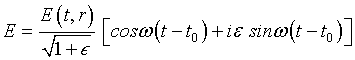(5)

Where ε defines the light polarization (ε = 0 for linear polarization) and E(t, r) is the envelope of the field. In a classical picture adopted here the motion of an electron is set by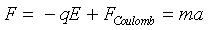(6)

Where “m” is the electron mass, “a” is the electron acceleration and FCoulomb is the Coulomb force that is time and space dependent. For the purpose of this paper the latter interaction is neglected. If we accept that the electron leaves the tunnel with zero velocity , there will be a distinct difference between velocity tunneled electrons of circularly polarized versus linearly polarized light: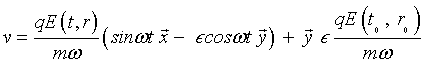(7)

Where vd=qE (t0,r0)/(mω) is the drift velocity that the electron acquires in ionization with circularly polarized light. This drift velocity stays with the electron long after the laser pulse is gone. In the absence of electron re-collision, which appears to be negligible for intensities of IR filament at atmospheric pressure , the tunneled electrons with linearly polarized light have negligible velocity in the plane of polarization, whereas tunneled electrons with circularly polarized light have the drift velocity vd. This drift velocity corresponds to c/100 for the maximum field in the filament.

The radiation of a non-relativistic moving charge  is expressed as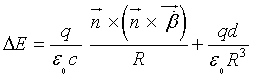(8)

where β=v/c , ε0 is the vacuum permittivity, is the unit vector of the observation point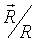and “d” is the displacement of the charge that can be calculated at time “t” from integration of the velocity given in Equation 7. Note that there are two terms in the electron response: the first one is the “radiation term” and the second the “dipole term”. For the condition of our study the radiation term is two orders of magnitude smaller than the dipole term and can be neglected. Note that the dipole term can be written as P/ε0 in which P=N (qd), where “N” is the density of the electrons. For a homogenous medium the index of refraction is calculated with “R” as the distance between particles. For mixed gases the contribution of each material (in the absence of interaction) can be calculated separately. In this paper the index of refraction is only calculated for electrons. The distance between electrons changes with time and position as given in Equation 4. The response of the electrons is a field ΔE, calculated for each point in space as a function of time, which modifies the applied field: E(z + Δz) = E(z) + ΔE. The radiated field ΔE is related to the traditional notion of index of refraction n(z, t) (no longer a constant) by the propagation equation written in the slowly varying envelope approximation and in retarded time: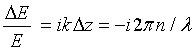(9)

Note that this approach is not restricted to a particular motion. If the medium is excited by multiple laser frequencies or existing nuclear and electromagnetic fields, they all contribute in the motion of the electron and therefore its radiation. In this paper the emphasis is on calculation of a collision-less electron response of ΔE.

The response due to the dipole radiation of the electrons at position “r” is calculated by time integration of Equation 7 and inserted in the dipole term of radiation equation Equation 8.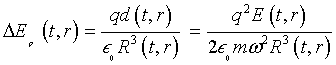(10)

In which E(t, r) is the laser field. Note that the dipole radiation exists only during the laser pulse. In this particular case the radiation of the moving electron agrees with the Drude model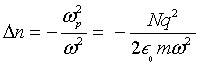(11)

If one considers a time dependent plasma frequency ωp that depends on the density of electrons “N” at each instant. Note that in a general case the motion of an electron is influenced by existing electromagnetic fields, collisions and Coulomb forces, therefore the refractive index of electrons cannot be defined solely by the density.

Tunneled electrons with circularly polarized light withhold a drift velocity (Equation 7) that is determined by the field value at the moment of ionization. The spiral motion of the tunneled electrons results in generating an expanding sphere in time. The electromagnetic fields in the presence of moving matter are related through Maxwell’s equations, suitably modified to include the effects of motion upon the electric and magnetic properties of matter . We assume that the expanding electron sphere in time has the constituent parameters of free space (μ= μ0 and ε = ε0 ).Let us assume that the expanding electron sphere is a perfect conductor with the field zero for r<b, where “b” is the radius of the sphere. One relation is necessary to complete the set of basic equations, which is Ohm’s law for a perfect moving conductor

E + v ×μ0 H = 0     (12)

Here “v” is the velocity of a macroscopic element of volume of the moving conductor. The solutions of Maxwell’s equations inside and outside the expanding sphere have to be matched across a moving surface. Due to the requirement of regularity at infinity, the problem is defined only by the magnetic vector potential “A”.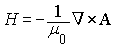(13)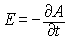(14)

and is the solution of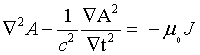(15)

Where “J” is the electric current density. Using the Green’s function, the field at distance “r” from the center of a sphere moving with constant velocity  is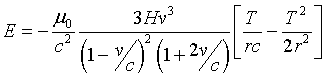(16)

Where “T=t − r/c”. In the case of tunneled electrons with circularly polarized light “r = R” is the distance between the electrons, “v” is the expansion velocity of the sphere (the drift velocity qE (t0, r0)/(mω) in Equation (7) and “T = a/v − R/c” where “a“ is the radius of the sphere at a given time. The total response at each point in the beam cross section at a given time “t’“ is calculated by adding all the responses of expanding spheres from ‘the initial ionization time“ti =−∞” to the final observation time “tf = t’ “, weighted by the probability of ionization at the moment of ionization “ti”.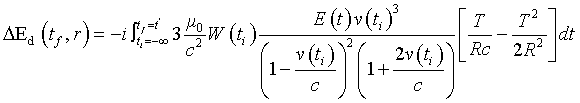(17)

The refractive index due to the expansion of electron spheres (Figure 1a) is compared to the dipole radiation (Figure 1b) with circularly polarized light. The difference between the two is presented in (Figure 1c). The dipole index is stronger in the first half of the pulse. The index due to the drift is stronger in the central portion of the beam profile and in later times of the laser pulse. Note that the two responses have 90°phase difference; dipole index is a real index and the drift index is an imaginary (absorption index). The drift index (imaginary) of tunneled electrons with linear polarization is another point of view in connecting microscopic effects such as light polarization to macroscopic effects in filaments can be seen through conservation of energy. In the strong field ionization  conservation of energy enforces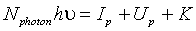(18)

Figure 1: a) Radiation field of expanding spherical conductors b) Dipole radiation c) Difference between Dipole radiation and radiation due to the drift motion neglected because of their low drift velocity and the index is mostly due to dipole radiation (real index).

Where “K” is the kinetic energy of the electrons and “N photon” is the number of absorbed photons in the ionization process. is the energy required to release an electron and Up is the ponderomotive energy due to the oscillation of electrons with the applied field. For the filament intensities the electron kinetic energy “K” of linearly polarized light can be neglected with respect to the energy of electrons with circularly polarized light . The plasma generated with circular polarization has higher energy (higher temperature).

From both perspectives of calculation of radiation of a moving charge and conservation of energy we come to the same conclusion that the index of refraction of weakly ionized gas is polarization dependent. Transient response of the ionized gas is calculated based on the equation of motion of the electrons. Other forces can be included to modify the electron motion. The same procedure can be applied to motion under the influence of multiple fields.

#### Acknowledgements

This work was supported by DTRA under grant number HDTRA1-11-1-0043, and by the Army Research Office, under the MURI grant W911NF-11-0297.

#### References

Select your language of interest to view the total content in your interested language

### Article Usage

• Total views: 12052
• [From(publication date):
June-2014 - Jan 23, 2020]
• Breakdown by view type
• HTML page views : 8259Can't read the image? click here to refresh